# The MathMap Language Tutorial

MathMap is a language for transforming existing images and producing entirely new ones. Think of it as the ultimate image and animation filter. This flexibility, however, comes at a price: Using MathMap to create a new transformation isn't as simple as using some pre-built image manipulation filter. Instead, you have to precisely describe what MathMap should do. This usually requires a bit of math knowledge (for most tasks, high-school math is more than sufficient) and it is necessary to know the MathMap language.

This document is a gentle introduction to the MathMap language. Very little mathematical knowledge is assumed, and almost no programming skills are needed--although they would certainly come in handy.

Please take the time to read through this introduction. Try out the examples we give and play around with them. Change them a little and see what happens. That way, you will quickly gain a feeling for what you can achieve with MathMap and in which ways. If you do this, we are confident that you will soon create your own image filters and maybe even get hooked on MathMap.

This tutorial deals with the following topics:

• Basic Principles
• The Cartesian Coordinate System
• Input Images
• The Polar Coordinate System
• Conditionals
• Variables
• User Values
• Animations
• ## Basic Principles

The basic operating principle of MathMap is very simple. To create an image of a given size, MathMap simply goes through all the elements (pixels) of the image to be created and "asks" your filter how the pixel in question should look like, i.e. what color it should have.

Let's make a filter that produces a white image:

```filter white ()
grayColor(1)
end
```

As you can see, MathMap filters always start with the word filter and end with the word end. The word white after filter is simply the name of the filter and you are free to choose your own names as you like. The parentheses () serve a purpose which we'll come to later.

grayColor is a function producing a gray level color. What it needs to know is the gray level you want to produce. In this case, the gray level we want is 1, which stands for white. 0 is black, and 0.5 is halfway in between. If you provide a value greater than 1, MathMap will use 1 instead (there is no color whiter than white!). Similarly, 0 will be used if a value less than 0 is passed to the function.

Such values given to functions are called arguments. As we have just seen, grayColor takes exactly one argument. Arguments are always given to a function after its name, enclosed in parentheses. As we will shortly see, if a function takes more than one argument, those arguments are separated by commas.

Producing gray levels is fun, but we'd like to play around with "real" colors, too. So, let's produce a red image:

```filter red ()
rgbColor(1, 0, 0)
end
```

As you can see, rgbColor takes three arguments and produces a color. Its first argument is the amount of red in the color. The second argument is the color's green component, and the third argument specifies the blue component. Again, useful values range from 0 to 1. Values too large or too small are clipped to 1 or 0, respectively. Try to change the values and see how it affects the output color.

## The Cartesian Coordinate System

So far, the pixels in our images have always had the same color. When we produce images with multiple colors, we usually want to determine the color based on the position of the pixel in question.

MathMap allows access to the coordinates of the pixel being calculated. It supports two coordinate systems. The first one, which you are certainly familiar with, is the cartesian coordinate system. Each pixel has two coordinates, called x and y. The following figure illustrates the cartesian coordinate system: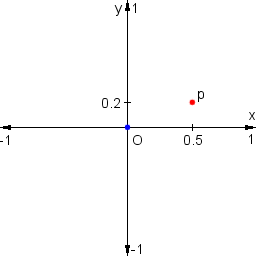The point labeled "O" is the origin of the coordinate system. Both its coordinates (x and y) are zero. The origin is always in the center of the image. The point "p" in the illustration has a value of 0.5 for the coordinate x and 0.2 for y. That's because it's halfway from the origin to the right edge of the image and one fifth to the top edge. As you can see, the x coordinate is 1 at the right edge of the image and -1 at the left edge. The same holds for the y coordinate and the top and bottom edges, respectively.

Let's use this knowledge to produce an image which is black on the left, white on the right and has gray levels in between. We want to produce this image: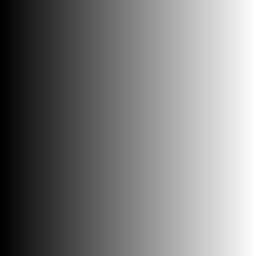We know that we can use grayColor to produce a gray level. However, we need a number between 0 and 1 to get colors between black and white. Let's look at what we have. At the right edge of the image the value of x is 1 and at the left edge it's -1. In order to get 0 at the left edge, we can simply add 1 to x, i.e. use x+1. At the right edge, however, we now get 2 instead of 1, which we can rectify by dividing by 2, which gives us (x+1)/2. Now we have what we want, and we can give this expression as an argument to grayColor:

```filter gray_gradient ()
grayColor((x + 1) / 2)
end
```

Try to do the same for the y coordinate, i.e. make a gradient from bottom to top instead of from left to right. You could also try to combine both coordinates to produce a gradient which goes from the bottom left corner to the top right corner.

## Input Images

While it's fun to produce completely new images, it is often nice or necessary to modify existing ones. We will use this as our input image:

What we have to do is tell MathMap that we want to give it an input image, and we have to give it a name, because there might be more than one. That's where the parentheses after the name of the filter come in. Between them, we give all the inputs our filter gets:

```filter ident (image in)
in(xy)
end
```

A few things are new here. Notice the declaration of the input image, which we call in. The reason why we have to specify with the word image that it's an image is that there are input types other than images, which we'll come to later.

What's new as well is the way we use the input image. It looks exactly like using a function with one argument, in this case xy, which we haven't explained yet, either. xy is a variable that combines x and y in one value, with the additional information that it's cartesian coordinates. That information is necessary because, as we'll see later, MathMap supports another coordinate system, as well.

So, you can use an input image just like you use a function. It takes one argument, namely the coordinates of the pixel that you request. In the script above, we are simply passing along the coordinates that our filter is given, so we just copy the input image, which is not very exciting.

A very simple effect is to flip the image horizontally. This can be achieved by changing the sign of the x coordinate, i.e. making negative coordinates positive and vice versa:

```filter flip (image in)
in(xy:[-x, y])
end
```

There's two other new pieces of syntax here. First of all we're using square brackets to combine the x and y coordinates into one value, called a "tuple". You can build tuples with as many numbers as you like. Tuples can only contain numbers, though, and not other tuples. The variable xy that we've seen in the filter ident above is a tuple, as well.

In addition, we're using the colon to give the tuple a so-called "tag", namely xy, which actually has no direct relation to the variable xy seen above. This specific tag says that the tuple is a pair of cartesian coordinates. Without that tag, MathMap wouldn't know which coordinate system you're using to request a pixel from the input image.

Try out the script for yourself. Also, try to predict what would happen if you changed the sign of the y coordinate instead, then try it out and see if you were right.

Now, let's shake the waves with our image. The function sin will come in handy for our purposes. This is what its graph looks like (by the way, this graph was produced by MathMap, using an expression by Hans Lundmark):As you can see, the value of sin oscillates between -1 and 1. The length of its oscillation period (the distance it needs to make a whole "cycle") is 2*pi. The value of pi, as is well known, is about 3.14159.

We will now try to shift whole pixel columns up and down, depending on their x coordinates. The shift pattern will resemble the graph of the sin function, only that we will use a period length of half the width of the image, i.e. 1, and we will shift them up or down by at most 0.1:

```filter sine_finn (image in)
in(xy:[x, y + 0.1 * sin(x * (2*pi))])
end
```

The resulting image looks like this:## The Polar Coordinate System

In addition to the familiar cartesian coordinate system, MathMap also supports the polar coordinate system. Each pixel has two polar coordinates, namely r and a. The following illustration helps in understanding the polar coordinate system: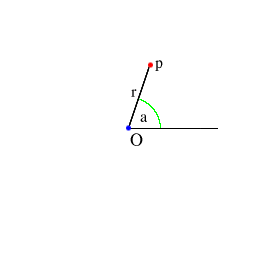The value of r is simply the distance from the origin (i.e. the center of the image) to that pixel.

The value of a is the angle between the positive x-axis and the line from the origin to the pixel in question.

However, the angle is not measured in degrees (0-360), but in radians, which range from 0 to 2*pi. This may seem a bit awkward, but it is more convenient mathematically. MathMap provides two functions to convert between radians and degrees, namely rad2deg and deg2rad.

Polar coordinates make it very easy to generate pond-like effects. When we try the wavy script from above and use polar instead of cartesian coordinates, leaving the a coordinate unchanged and shifting the r coordinate, we get the following expression:

```filter finn_pond (image in)
in(ra:[r + 0.1 * sin(r * (2*pi)), a])
end
```

which generates this image:Notice how this script uses the tag ra, instead of xy, to let MathMap know that the coordinates given to the input image are polar and not cartesian.

## Conditionals

Let's create an image which looks like a shooting target:Obviously, whether a pixel is red or white depends solely on its distance from the center, which we know is available as r. I have chosen the width of each ring as 0.2, i.e. the distance between the radii of the inner circles of two neighboring red (or white) rings is 0.4. Hence, the expression we are looking for is periodic with a period of 0.4.

To solve this problem, we will use the modulo operator, which is available as %. Its value is the remainder of the division of its left operand by its right operand. As an example, 7%3 is 1 because the remainder of the division of 7 by 3 is 1. This operation is periodic. Its period is the value of its right operand (the divisor). Furthermore, the result of the operation is never greater than the right operand. So, for example, r%0.4 is periodic with a period of 0.4 and is always between 0 (inclusive) and 0.4 (exclusive). Let's see what this looks like:

```filter rmod ()
grayColor((r%0.4)/0.4)
end
```

In order to be nice to grayColor, the value is scaled to be in the range from 0 to 1 (instead of 0.4). The resulting image looks like this:You can see that the value starts out as 0 at the center of the image, climbs to 1 at a distance of 0.4 from the center and then immediately drops to 0 again, repeating this cycle forever (well, in our case, to the boundaries of the image).

You may want to try to leave out the rescaling "/0.4" to see the difference.

All we have to do now is to check whether we are in the first half of a period (in which case r%0.4 is less than 0.2), or in the second. If we are in the first, the color for the pixel is red, otherwise it is white. MathMap provides a construct for such decisions:

```filter target ()
if r%0.4 < 0.2 then
rgbColor(1,0,0)
else
rgbColor(1,1,1)
end
end
```

The indentation is used merely to make the expression easier to read. You can indent your code any way you like (or not at all).

The expression should be easy enough to understand. If the condition is fulfilled, the result is the color red, otherwise it is the color white.

## Variables

Sometimes you want to use one value in multiple places in your expression. It's not necessary to write that value twice. Instead you give it a name by which you can refer to it later. Let's say we want to produce an image like this:As you can see, the pixels from the original image fade to black with the distance from the center. They reach the black color at the corners of the image.

The variable r, which holds the distance from the center of the image, is measured in the same distance units as the cartesian coordinates, and its maximum value, which it reaches in the four corners of the image, is provided by the constant R (which is the square root of 2 in square images, in case you must know). If we scale this down to 1, it's much easier to work with, so we'll use r/R. This expression's value is 0 at the center of the image and increases with the distance from the center. It reaches 1 in the corners, exactly where we want the color to be solid black.

Given the color of a pixel in the original image and its transformed (as above) distance from the center, we can now figure out what to do. If the transformed distance is 0 (in the center) we want the original color unchanged. If it's 1, we want the color black. We can reach that effect by multiplying the three color components of the pixel by 1 minus the transformed distance, i.e. by 1-r/R.

We can use the functions red, green, and blue to access the components of a color. Now we could write the red component of our output image as red(in(xy))*(1-r/R). We'd have to use equivalent expressions for the green and blue components and then use them as arguments to rgbColor. By assigning the values in(xy) and 1-r/R to variables, which we'll call p and d (you can choose any name you like, as long as it's not the name of a built-in constant or variable or a keyword; consult the MathMap reference for the names of all of those), we can write the resulting expression much shorter as rgbColor(red(p)*d, green(p)*d, blue(p)*d). The complete filter is:

```filter finn_vignette (image in)
p=in(xy);
d=1-r/R;
rgbColor(red(p)*d, green(p)*d, blue(p)*d)
end
```

As you can see, assigning values to variables is very straightforward. You must separate variable assignments and other expressions with semicolons.

By the way: Using more advanced features of MathMap you can write an expression equivalent to the above as

```filter finn_vignette (image in)
lerp(r/R, in(xy), grayColor(0))
end
```

## User Values

Sometimes you need to put some values into your script which are more or less arbitrary. Often you want to try out several different values, and it's tedious to change the script every time by hand. That's where user values come in. Let's reiterate our wave example:

```filter sine_finn (image in)
in(xy:[x, y + 0.1 * sin(x * (2*pi))])
end
```

There are two parameters here which we have chosen more or less arbitrarily, namely the amplitude of the wave (in this case 0.1) and the wave length (in this case implicitly given as 1). Wouldn't it be nice if we could change these values with sliders instead of having to edit the expression?

That's where inputs come in again. We've seen above that we can give images as inputs to filters. Now we'll learn how to supply numbers as well:

```filter sine_finn (image in, float amplitude: 0-2, float wavelength: 0-10)
in(xy:[x, y + amplitude * sin(x * (2*pi) / wavelength)])
end
```

The type float denotes a so-called floating point number, which represents a real number and can have a fractional component, in contrast to integers, which you can specify with the word int. After the name of the argument you can also give a range of allowed values, in the case of the argument amplitude above from 0 to 2.

There are not only argument types for images and numbers, but also for colors, color gradients and curves. Check out the Reference Manual for details.

## Tuples and Tags

Now it's time to look at a more technical subject, namely MathMap's type system. We've already talked about it a little when we examined coordinates, and now we'll go into a bit more detail.

The type system of MathMap is designed to be as invisible as possible, but in order to unleash MathMap's full potential, you will need to know one or two things about it. Don't worry, it's not very complicated.

Sometimes it's convenient to treat two or more numbers as a single value. One such example is colors. A single color is actually four distinct numbers. We have already come across three of them, namely the red, green, and blue components. The fourth is the color's transparency value, called alpha. A color with an alpha of 1 is completely opaque, like all the colors we have seen so far. An alpha component of 0 means full transparency, 0.5 means half transparent, and so on.

So far, we have always treated colors as single values. We have constructed colors using functions such as rgbColor and retrieved their components with functions like red. We can, however, do these things without using those functions. This, for example, is the half-transparent color green:

```rgba:[0, 1, 0, 0.5]
```

One or more numbers within square brackets, separated by commas, constitute a tuple. So, tuples are just ordered collections of numbers. They are ordered because MathMap remembers in which order you have written their components. For example, the tuple [1,2,3] is not the same as [3,2,1].

The name rgba is a tag. The tag rgba tells MathMap that that tuple is not just four numbers, but a color with red, green, blue and alpha components. This begs the question whether there are other kinds of colors. Actually, there are. MathMap also supports colors in the so-called HSV color space. Those colors are given the tag hsva. MathMap uses the tags to determine how to interpret the numbers in the tuples.

Many operators and functions work on tuples as well as on single numbers. The functions min and max for example, calculate the minimum and maximum values for each pair of tuple elements. To set the red component of an image to 0, for example, you can use the following script:

```filter remove_red (image in)
min(in(xy), rgba:[0, 1, 1, 1])
end
```

## Animations

MathMap provides the functionality to create animations. To that end, the language provides a variable called t. For each single picture in the animation (such pictures are called frames) t has a different value, depending on the position of the frame within the whole animation. The first picture in the image always has t set to 0, while for the last picture it is set to 1. Actually, the latter statement isn't always true, as we will discover shortly, but for the time being, simply assume that it is.

The following script produces an animation which fades from black to white:

```filter black_to_white ()
grayColor(t)
end
```

You will often want to produce animations which loop seemlessly, i.e. which look like one endless animation when looped. For such animations, make sure that the image with a t value of 1 looks exactly like the one with a value of 0, like in the following script:

```filter black_white_loop ()
grayColor((sin(t * 2*pi) + 1) / 2)
end
```

The problem here is that if MathMap would render the first image in the animation with t as 0 and the last image with t as 1, you would have the same frame twice when looping. Therefore, MathMap lets you choose (in the user interface) whether you would like to create a periodic (looping) animation or not. If you do, t never reaches 1 at the end of the animation but stops shortly before, depending on how many frames you want your animation to have. For example, for a periodic animation with 10 frames, t takes on the values 0, 0.1, 0.2, ... 0.9.

Hint: One way to make periodic animations is to use periodic functions like sin, cos or the modulo operator %.

## Some Useful Functions

Here is an overview of some MathMap functions which are very useful in many situations. This is not a complete function reference. If you are looking for one, you'll find it in the Reference Manual.

### scale

Quite often you find that you have a value which varies within a given range, but you want the range to be different. Take, for example the gray gradient:The variable x varies from -1 to 1 but you want it to be between 0 and 1. In such cases you can use the scale function. The expression scale(x, -1, 1, 0, 1) is 0 when x is -1 and 1 when x is 1. Hence, you can create the above image with the script

```filter gray_gradient ()
grayColor(scale(x, -1, 1, 0, 1))
end
```

### lerp

Suppose we want to produce a gradient from red to green: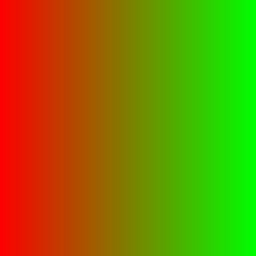We know from above that we can use scale(x, -1, 1, 0, 1) for a value which is 0 at the left image edge and 1 at the right edge. lerp does the rest: it takes two tuples and produces a value which is "in between" these two values by the same amount as its first argument is in between 0 and 1. Hence, the gradient above can be produced by this expression:

```filter redgreengradient ()
lerp(scale(x, -1, 1, 0, 1), rgbColor(1,0,0), rgbColor(0,1,0))
end
```

### inintv

The function inintv makes it easy to check whether a value lies within a given range. inintv has a value of 1 if the condition is fulfilled, and of 0 otherwise. You can use this as a condition in an if expression, or as a value in its own right. For example, this script draws a white ring with an inner radius of 0.4 and an outer radius of 0.6:

```filter ring ()
grayColor(inintv(r, 0.4, 0.6))
end
```

### clamp

Sometimes you have values which you want to lie within a given range. In case they don't, you simply want them to take on the largest value within the range, if they are too large, or the smallest if they are too small.

MathMap often does such operations automatically, for example if you produce colors with components larger than 1 or smaller than 0.

If you have to do it yourself, clamp can help you. For example, clamp(v, [0,0,0], [1,1,1]) restricts every element of v to be in the range from 0 to 1.

### rand

The function rand generates a random number. It takes two arguments: The minimum and the maximum value of the number to be generated. This filter, for examples, randomly scatters the pixels of the input image (but not more than a distance of 0.05 away from their original location in both directions):

```filter scatter (image in)
in(xy:[x + rand(-0.05,0.05), y + rand(-0.05,0.05)])
end
```

### noise

In image manipulation, one often needs a function which is random but doesn't change as abruptly as rand does. noise is a so-called solid noise function. It takes a tuple of three numbers and returns a value between -1 and 1. If the input arguments change only by a little, so does the resulting value. The overall "look" of the function is random, though. It's hard to describe, so it's best you see for yourself:

```filter noise_demo ()
grayColor(scale(noise([x*5, y*5, t*2]), -1, 1, 0, 1))
end
```

As you can see, the third input value depends on t, so try out changing t. For t being 0, the resulting image looks like this: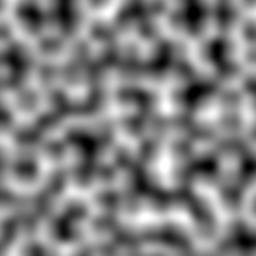## Further Information

This tutorial has, despite its length, not covered all features and details of MathMap. For example, we didn't even mention loops (a programming language feature having nothing to do with animations).

To get more detailed information about the MathMap language, see the MathMap Reference Manual.

A very good way to learn how to do things with MathMap is to look at the examples supplied with it. Pick the examples you find interesting, look at the filter sources, and try to figure out how they work.

You might also want to look at the MathMap Homepage for announcements, new documentation or interesting links. The best way to get involved in the MathMap community is by joining the MathMap Google Group.

If you like MathMap, or if you have suggestions or questions regarding the MathMap language or user interface, feel free to contact the author. I am looking forward to your feedback.